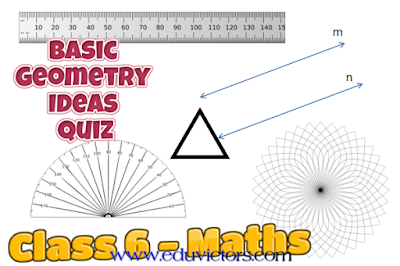## Basic Geometry Ideas - Quiz

Class 6 - Mathematics

Q1: Three or more lines which pass through same point are called

(a) intersecting lines
(b) parallel lines
(c) perpendicular lines
(d) concurrent lines.

Q2: Geometrical figure which has no dimension is

(a) line
(b) plane
(c) line segment
(d) point.

Q3: The lines which do not intersect and have equal distance between them are called:

(a) parallel lines
(b) perpendicular lines
(c) intersecting lines
(d) straight lines

Q4: Number of points a line can have are:

(a) infinite
(b) one
(c) two
(d) zero.

Q5: The point at which two lines cross each other is called:

(a) point of intersection
(b) point of concurrence
(c) parallel lines
(d) concurrent lines.

Q6: A line segment has:

(a) definite length but no end points
(b) infinite length but no end point
(c) definite length and have end points
(d) none of these.

Q7: If the length of a line segment AB = 3 cm then 2AB will be

(a) 8 cm
(b) 6 cm
(c) 4 cm
(d) 9 cm

Q8: Number of line segments which can be drawn joining two points:

(a) two
(b) one
(c) infinite
(d) none

Q9: Two line segments having the same length are said to be:

(a) same
(b) unequal
(c) parallel
(d) equal

Q10: The number of diagonal in a triangle are:

(a) 3
(b) 2
(c) 0
(d) 1

1: (a) intersecting lines
2: (d) point
3: (a) parallel lines
4: (a) infinite
5: (a) point of intersection
6: (c) definite length and have end points
7: (b) 6 cm
8: (b) one
9: (d) equal
10: (c) 0